Examples

Serial order wise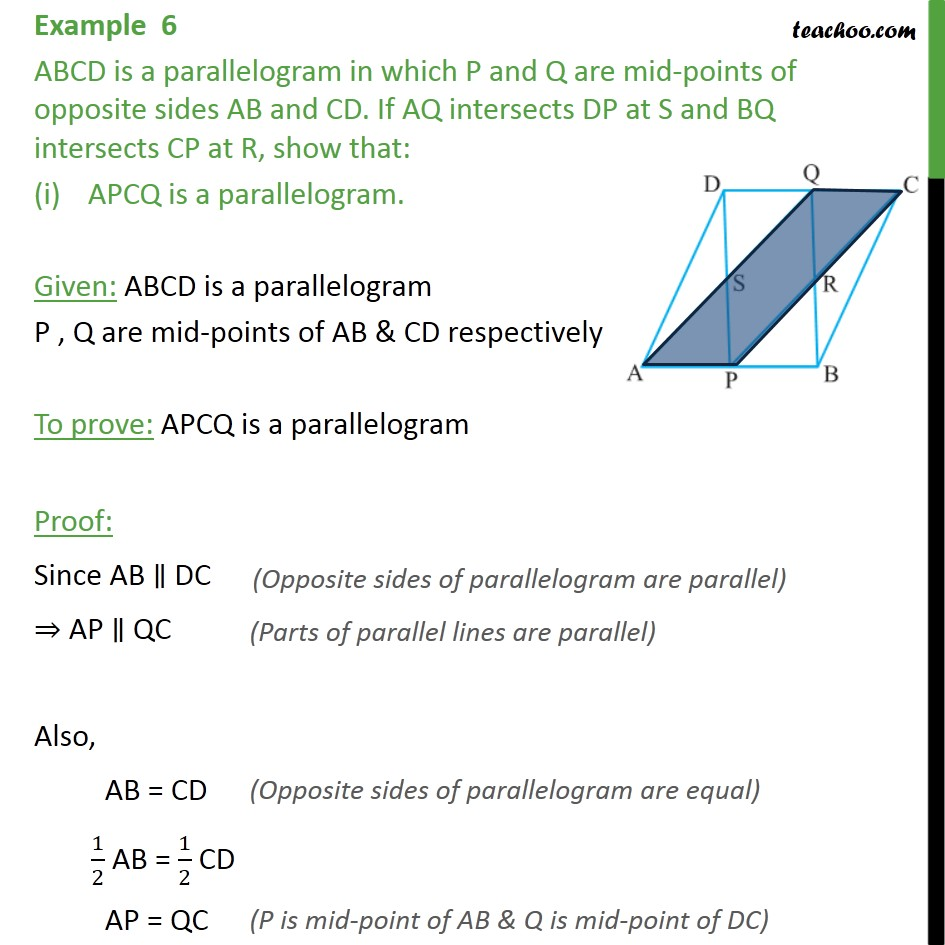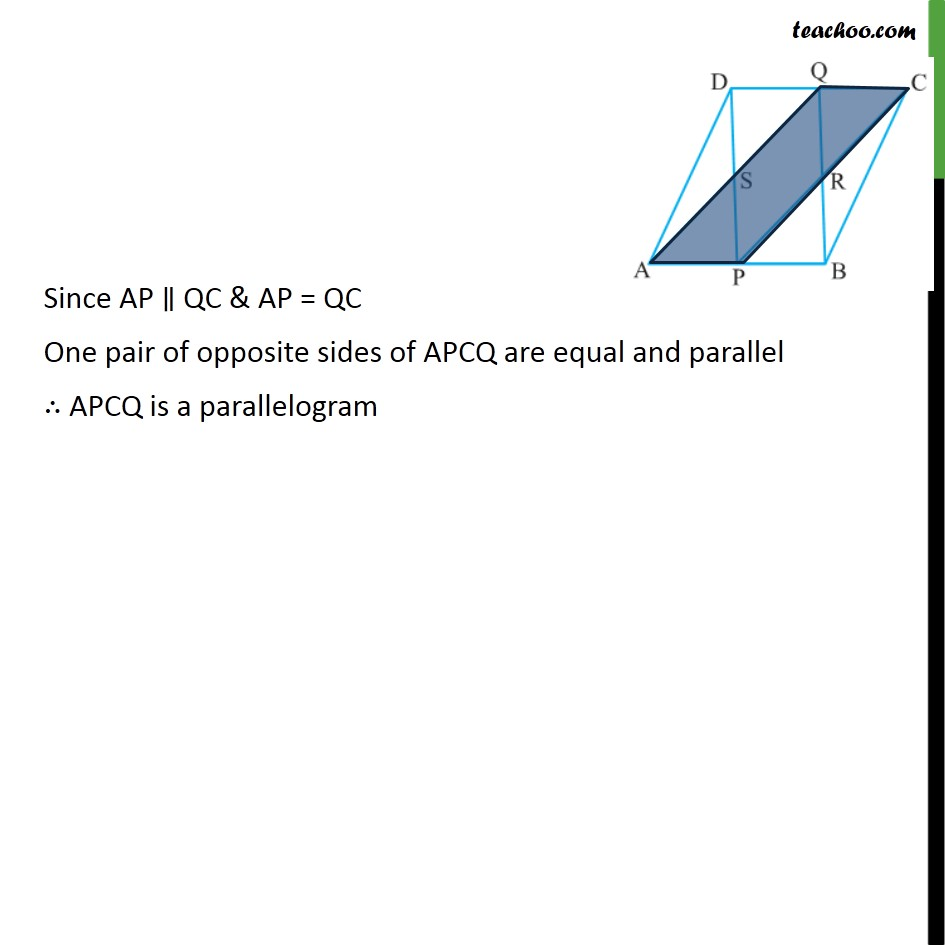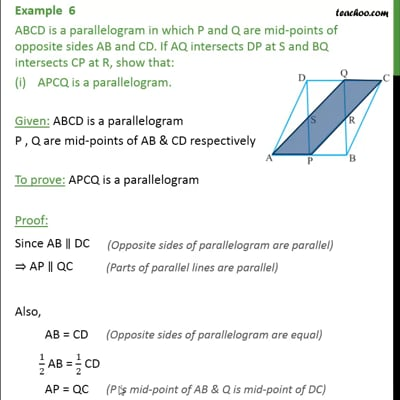This video is only available for Teachoo black users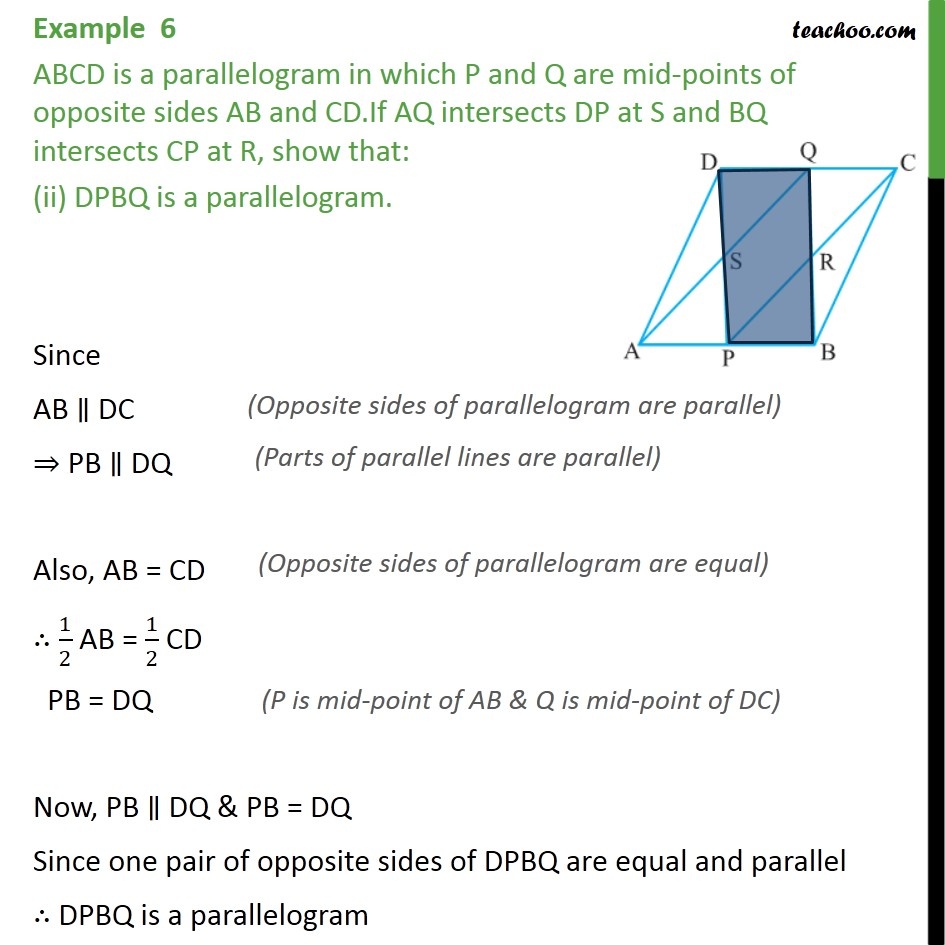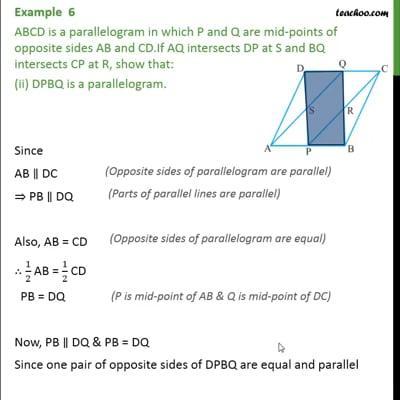This video is only available for Teachoo black users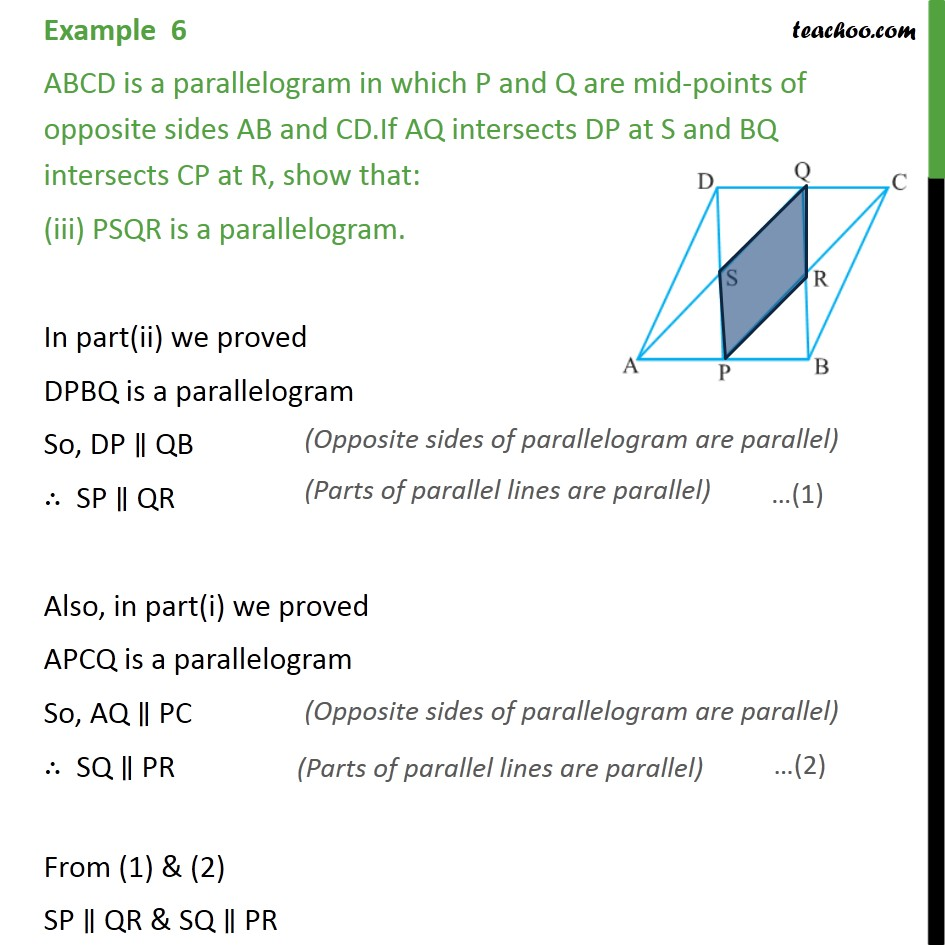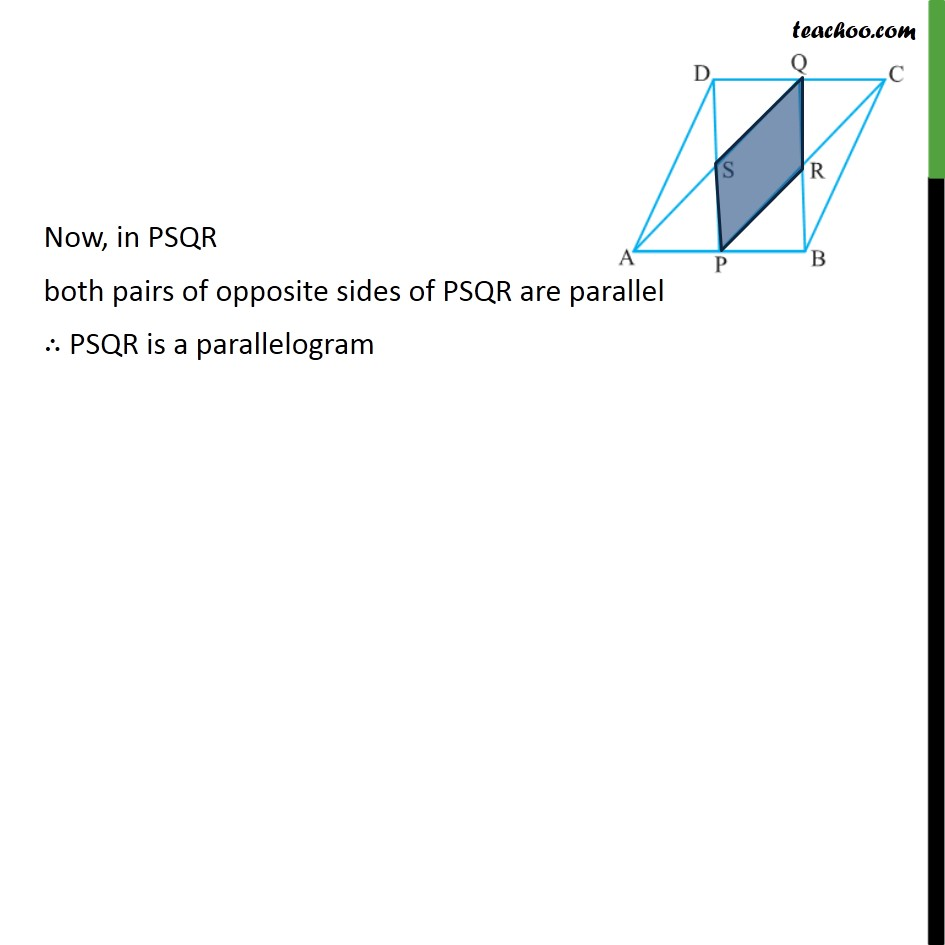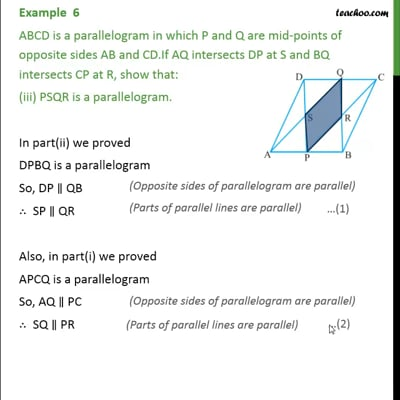This video is only available for Teachoo black users

Maths Crash Course - Live lectures + all videos + Real time Doubt solving!

### Transcript

Example 6 ABCD is a parallelogram in which P and Q are mid-points of opposite sides AB and CD. If AQ intersects DP at S and BQ intersects CP at R, show that: APCQ is a parallelogram. Given: ABCD is a parallelogram P , Q are mid-points of AB & CD respectively To prove: APCQ is a parallelogram Proof: Since AB ∥ DC ⇒ AP ∥ QC Also, AB = CD 1/2 AB = 1/2 CD AP = QC Since AP ∥ QC & AP = QC One pair of opposite sides of APCQ are equal and parallel ∴ APCQ is a parallelogram Example 6 ABCD is a parallelogram in which P and Q are mid-points of opposite sides AB and CD.If AQ intersects DP at S and BQ intersects CP at R, show that: (ii) DPBQ is a parallelogram. Since AB ∥ DC ⇒ PB ∥ DQ Also, AB = CD ∴ 1/2 AB = 1/2 CD PB = DQ Now, PB ∥ DQ & PB = DQ Since one pair of opposite sides of DPBQ are equal and parallel ∴ DPBQ is a parallelogram Example 6 ABCD is a parallelogram in which P and Q are mid-points of opposite sides AB and CD.If AQ intersects DP at S and BQ intersects CP at R, show that: (iii) PSQR is a parallelogram. In part(ii) we proved DPBQ is a parallelogram So, DP ∥ QB ∴ SP ∥ QR Also, in part(i) we proved APCQ is a parallelogram So, AQ ∥ PC ∴ SQ ∥ PR From (1) & (2) SP ∥ QR & SQ ∥ PR Now, in PSQR both pairs of opposite sides of PSQR are parallel ∴ PSQR is a parallelogram